# 11 – Root of the Problem

 < ρℝεν | ℂσητεητs | ℕεχτ >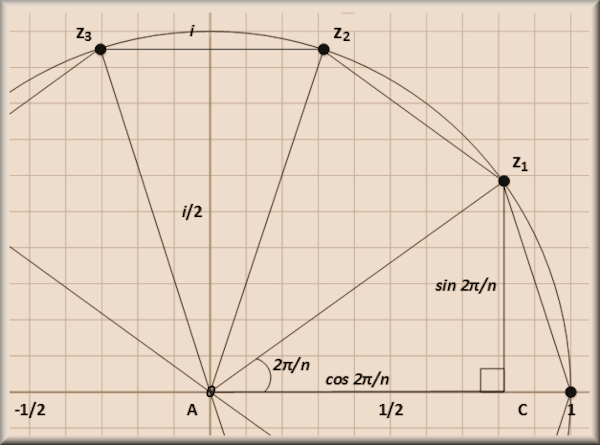“The future has many names: For the weak, it means the unattainable. For the fearful, it means the unknown. For the courageous, it means opportunity.” – Victor Hugo

Looked at from the perspective of today’s Mathematicians (and Physicists) Group Theory and Symmetry are conjoined. It is hard to talk about one without referencing the other, as reflected in the title of this book. However what we now call Group Theory emerged in the 1800s from very different considerations and very different ways of doing Mathematics; ones that – at least at first sight – are many miles from the concept of Symmetry, namely the roots of Polynomial Equations. Writing a book about Group Theory without taking about the roots of polynomials would render the tome incomplete and the reader blissfully unaware of the early history of the field. In in Chapter 2 of this book, I introduced Groups via a technical definition. In in Chapter 3, I went on to describe geometric examples. However the true genesis of Group Theory is neither in its modern day definitions, nor in geometry. Instead the subject came about because of the efforts of Mathematicians to solve equations. Given this, solving equations is the subject of the next two Chapters.

Many Names, Many Numbers

As referenced in a footnote in Chapter 7, a polynomial (from “many names”) is an algebraic expression of the form:

anxn + an-1xn-1 + … + a2x2 + a1x + a0 = 0

Where the ai, with i ranging from 0 to n, are called the coefficients of the equation and often are set to be Rational Numbers (ai ∈ ℚ), or sometimes just Integers (ai ∈ ℤ).

x, which is raised to powers ranging from 0 to n  is called the unknown.

The general idea of a Polynomial is to work out what value (or values) of the unknown, x, satisfy the equation. What value of x can I plug in to the left hand side in order to ensure that this indeed equals 0? Solving such Polynomials is of practical importance in many applications.

The degree of a polynomial is the highest power of the unknown x that appears in it. If the highest power is x2 (x × x) then we say that the degree is 2. If the highest power is x7 (x × x × x × x × x × x × x) then we say that the degree is 7. Polynomials of some small degrees have special names:

 Degree Highest Power Name 1 x (or x1) Linear 2 x2 Quadratic 3 x3 Cubic 4 x4 Quartic 5 x5 Quintic

Quadratic Polynomials (aka Quadratic Equations) are a staple of school Mathematics. Students learn that they have two roots (values of x which yield the result 0). Sometimes these may be obvious as in:

x2 + 2x – 8 = 0

Which can be factored in to:

(x – 2)(x + 4) = 0

And we can see that when x = 2 the terms in the first bracket sum to zero and when x = -4 the terms in the second bracket sum to zero. In either case 0 times anything is 0 so the equation works. This means that the roots are x = 2 and x = -4.

Not all factoring is so easy to see and the same scholars will later be presented with a general formula for working out the roots of a Quadratic. Any Quadratic Polynomial may be written as:

ax2 + bx + c = 0

So the coefficients are a, b and c

In this case the roots can be calculated in terms of the coefficients using the following generic formula:

(-b ± √(b2 – 4ac))/2a

The ± symbol relates to the two roots of a Quadratic and saves time by showing these in the same formula. More explicitly the two roots are:

(-b + √(b2 – 4ac))/2a

and

(-b √(b2– 4ac))/2a

If we look at the example we solved by factoring above:

x2 + 2x – 8 = 0

We can check that the general formula for evaluating roots works by setting a = 1, b = 2 and c = -8. Working out the first root we get:

(-2 + √(22 – 4 × 1 × -8))/(2 × 1)

= (-2 + √(4 + 32))/2

= (-2 + √(36))/2

= (-2 + 6)/2

= 4/2 = 2

Which gives us the x = 2 root.

Going back to the penultimate step in the evaluation of the formula and replacing + 6 with – 6 gives us:

(-2 – 6)/2

-8/2 = -4

Which is of course the other root, x = -4.

Over time, Mathematicians worked out similar but more complex formulae that provided general ways to work out the roots of the Cubic and the Quartic Equations (Polynomials of degree 3 and degree 4).

Here it is worth pausing to note (as also mentioned in a footnote in an earlier Chapter) that the number of roots of a Polynomial is equal precisely to its degree. Thus Cubic Equations have three roots, Quartic four and so on.

Despite the progress made in determining formulae for the Cubic and Quartic, a general formula for solving Quintic Equations remained elusive. It is in this last activity that we are most interested here and we will return to talk about it in the next Chapter. In this Chapter, we will pursue what may initially seem like a diversion to look further at the properties of Complex Numbers. We will find however that this is a diversion which eventually brings a new perspective to aspects of this book’s eventual objective.

Roots of UnityIn Chapter 7 we met the number i which has the remarkable property that i2 = -1. The reader may recall that one motivation for the introduction of the concept of Complex Numbers (ones featuring i) was results that sometimes arose from just the type of general formulae we discussed in the previous section.

If we consider the Quadratic formula:

(-b ± √(b2 – 4ac))/2a

We can see that if the coefficients in ax2 + bx + c = 0 are such that 4ac is bigger than b2 then the number we need to take the square root of in the formula will be less than 0. This rather quotidian occurrence needs i to resolve it. An argument for Complex Numbers to be embraced is the requirement to be able to solve all Quadratic Equations with Rational coefficients.

Given the definitional property that i2 = -1, we can also note that:

(i2)2 = (-1)2

Or, using the well-established property of powers that (xa)b = xab:

i4 = (-1)2 = 1

This observation shows us that i is a root of the Quartic:

x4 – 1 = 0

A moment’s thought will tell us that –i is another root. If we combine these with the two more obvious solutions, 1 and -1, then we have the required four roots of a Polynomial of degree 4. Because the two equations:

x4 – 1 = 0

x4 = 1

are identical, {1, -1, i, –i} are called the fourth roots of unity (or roots of 1).

From Algebra to Geometry…

I also previously introduced a way of thinking about Complex Numbers called the Complex Plane. This is a two dimensional grid in which, for a generic Complex Number a + ib, the Real component, a, is plotted on the horizontal axis (customarily referred to as the x-axis) and the Imaginary component, ib, is plotted on the vertical axis (or y-axis). Let’s employ this approach to look at the fourth roots of unity.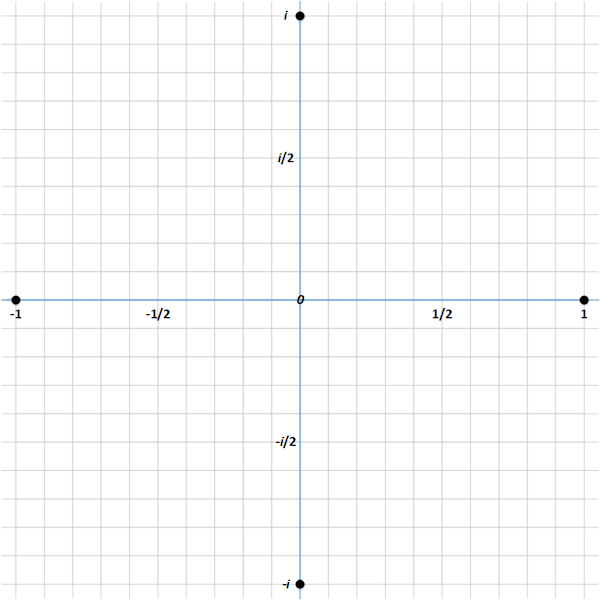They are rather nicely arranged (something that should have the attention of a Group Theorist piqued immediately) and a couple of geometric insights immediately come to mind.

First if we draw a circle with a radius of 1 and centred on the origin (0, or maybe more explicitly 0 + i0), then this passes through all four fourth roots of unity.Second if we join up the four roots using straight lines, we get a square touching the unit circle we have already drawn.Is this some sort of weird coincidence or is something deeper happening here?

Continuing with a geometrically inspired approach, what about if we create points in between each of the fourth roots of unity, still on the circle and join these up. The result is a regular octagon. What can we say about the new points we have created?

Well some elementary geometry tells us that the angles between the lines going to the four vertices of the original square are 90°. In order to create the octagon, we need to bisect these angles and 90°/2 = 45°. We can then work out the Real and Imaginary components of these new points using nothing more complex than Pythagoras’s Theorem.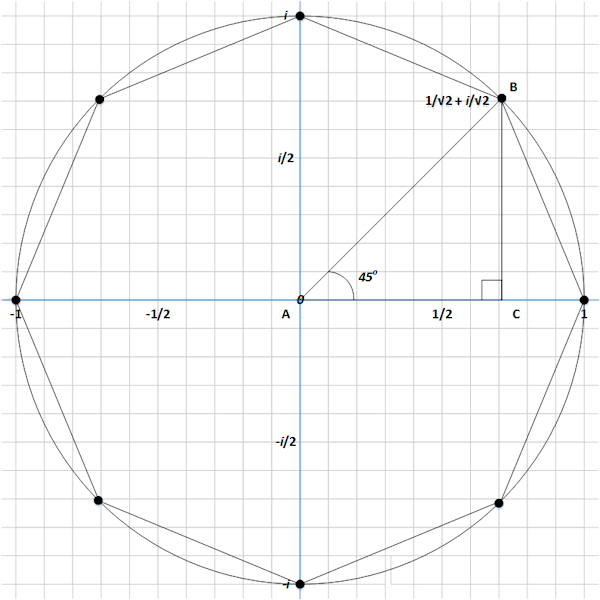As shown above, we can construct a right-angled triangle with the hypotenuse being the line AB (of length one) going from the origin (A) North East to the first of our new points (B), with the opposite side (BC) dropping from the new point to the x-axis and the adjacent side (AC) following the x-axis from the origin to where the opposite side intersects this (C) .

Our bisection tells us that the angle of this triangle next to the origin ∠BAC is 45° (as of course is the other one ∠ABC) and we know that 45° triangles are isosceles so we can immediately deduce that |BC| = |AC| and therefore that the Real and Imaginary components of our first new point have the same size.

By Pythagoras:

|AB|2 = |BC|2 + |AC|2

Or as |BC| = |AC|

|AB|2 = 2|BC|2

or

√(|AB|2/2) = |BC|

Noting that |AB| = 1 we have:

|BC| = |AC| = 1/√2

So the our new point B, when written as a Complex Number, equals:

1/√2 + i/√2

Is this a root of unity? As we have doubled the number of sides of our regular polygon (square = 4, octagon = 8) maybe we can explore what happens if we raise or new point to the same power as the number of sides of the shape that defines it. Let’s call this new point z then:

z2 = (1/√2 + i/√2)( 1/√2 + i/√2) =

1/2 + i/2 + i2/2 =

1/2 + 2i/2 – 1/2 =

i

Which is an interesting result as we already know that i4 =1.

Again using our result relating to powers:

z8 = (z2)4 = (i)4 = 1

So our newly constructed point is an eighth root of unity. We can see that the three other new points forming the octagon together with the vertices of the original square are given by:

1/√2 – i√2

-1/√2 + i/√2

and

-1/√2 – i/√2

If we add in our four fourth roots of unity (noting that if x4 = 1 then obviously x8 = 1 as well), we get the required 8 roots of a Polynomial of degree 8. Once more they have a nice symmetrical (note the used of the word) arrangement, all lying on a regular eight-sided polygon.

It’s probably evident that the above result would work if replicated for 16th roots (by bisecting the octagon’s angles to create a regular 16-gon with its vertices all on the unit circle). Indeed the 2n th roots of unity will be found at the 2n vertices of a regular 2n-gon all lying on the circle of unit size centred at the origin.

Is this just a phenomenon of powers of two? Let’s consider the next simplest example, the cube roots of unity, or roots satisfying the equation:

x3 – 1 = 0

(or equivalently x3 = 1)

If we consider the first equation above and note that x = 1, or x – 1 = 0 is the obvious first root, we can factor this out to get us:

(x – 1)(x2 + x + 1) 

So the other two cube roots of unity must be the roots of the Quadratic Equation:

x2 + x + 1

Using our handy Quadratic Formula we note that a = 1, b = 1 and c = 1. This gives us:

(-1 ± √(12 – 4 × 1 × 1))/(2 × 1) =

(-1 ± √(1 – 4))/2 =

(-1 ± √-3)/2 =

-1/2 + i√3/2 and -1/2 – i√3/2

Let’s double check our workings by cubing one of these:

(-1/2 + i√3/2)3 =

(-1/2 + i√3/2) (-1/2 + i√3/2) (-1/2 + i√3/2)

Working out the first two brackets we get:

(1/4 – i√3/4 – i√3/4 + i23/4) (-1/2 + i√3/2) =

(1/4 – i√3/2 – 3/4) (-1/2 + i√3/2) =

(-1/2 – i√3/2) (-1/2 + i√3/2)

Multiplying this result by the third bracket we get:

-1/4 – i√3/4 + – i√3/4 – i23/4 =

1/4 + 3/4 = 1

So our result holds. Given that our other complex cube root of 1 appeared magically in the calculations above as the square of the first complex cube root (an observation we will return to later in this Chapter), we can see that a symmetrical argument proves that the other number is also a cube root of 1).

Let’s plot these points on our complex plain.Again we have a nice symmetrical result an equilateral triangle with each vertex located on the unit circle. Applying a similar bisection–based approach to the one we employed with a square above, we can show that the sixth roots of unity are located at the vertices of a regular hexagon inscribed in a unit circle and that the 3n th roots of unity will be found at the 3n vertices of a regular 3n-gon all lying on the circle of unit size centred at the origin.

From Geometry to Trigonometry…

It does rather seem that a pattern is emerging here, but we are in the realm of Mathematics, not a looser discipline, and so leaping to state that:

The nth roots of unity all lie at the vertices of a regular n-gon inscribed in a unit circle centred at the origin

would be premature (though, as we will see, not inaccurate). We need to prove this statement instead of just offering some compelling examples. How to approach such a proof?

As with many areas of Mathematics, Trigonometry offers us a way forward. If our assertion above is correct, then when we consider the nth roots of unit, we need to draw n equally spaced lines all emanating from the origin. Further the first of these lines must go East along the x-axis. The vertices of the required n-gon are precisely where these n lines meet the unit circle. To construct these lines, we need to divide the total angle of the circle (360°) into n parts, so the angle between successive lines will be 360°/n. This looks something like the following: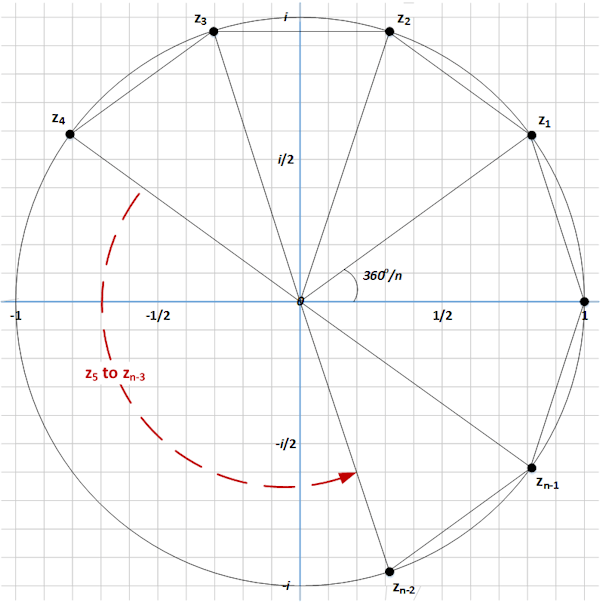I’m going to adopt the labelling scheme shown in the above diagram whereby our proposed n nth roots of unity, all lying at the vertices of a regular n-gon inscribed in a circle of unit radius centered on the origin, are denoted by {1, z1, z2, … , zn-1}, where the set proceeds anticlockwise. Hence z1 is the first nth root of unity after 1 moving in an anticlockwise direction; z2 is the second and so on.

To plug an actual example into this generic framework, when n = 4 we have 360°/4 = 90°, which is of course the square example we saw above. When n = 3, then 360°/3 = 120° and we have the triangle example from above. When n = 8, then 360°/8 = 22.5° and we have the octagon.

Let’s focus on the regular n-gon and see what general results we can prove. Before we do so, I need to shift our frame of reference slightly. I have to date been manfully using things like 90° to describe angles and this is the measurement system that is used most frequently in schools. For a lot of Mathematics, and this includes much of what we are about to explore, it makes sense to use radians to measure angles.

This switch is actually no different to using kilometres versus miles to measure the length of a journey. The physical reality is not changed, just our units of measurement.

While 1 mile is approximately 8/5 km, for degrees and radians, the conversion factor is precisely 180° = π radians. We can use this to can derive:

and so on…

This may seem an odd way of measuring angles but it actually emerges very naturally. We will have all learnt at school that if the diameter of a circle is d and its circumference is c, then:

c = πd

If we consider a circle of unit radius (remembering that a radius is half a diameter) then this equation becomes:

c = 2π and

c/2 = π

This is where the mapping of 180° = π radians comes from. What we are measuring is the length of a unit circle’s circumference that is swept out by a given angle: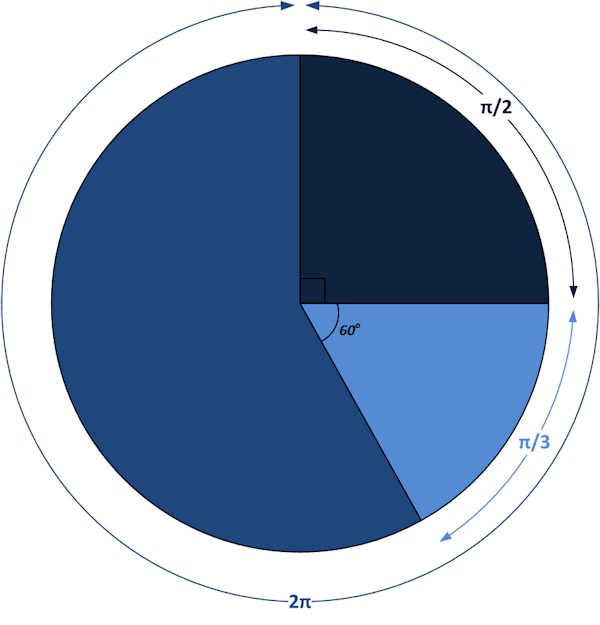So, to construct our regular n-gon, rather than the angle between each successive pair of our n lines being 360°/n, we will say that it is 2π/n. Believe it or not, this change in units of measure will help us later.

The first vertex of our regular n-gon is at 1 (or 1 + i0). Where will the second point (working anticlockwise) which we have labelled z1, be located? As when we constructed a triangle for the 45° (π/4 radians) case, we need to form a right-angled triangle, but this time we can’t rely on the simplifying result that this is isosceles, it won’t be. Instead we need to use those two stalwarts of Trigonometry, cosine and sine (abbreviated as cos and sin).

Cosine and Sine were both featured in a box at the end of Chapter 6, let’s pause to offer a refresher on these. Consider a generic right-angled triangle as in the figure below:Here the bottom left-hand angle has a value of θ, the hypotenuse has length a, the adjacent side has length b and the opposite side has a length of c. We then have the following definitions:

sin θ = c/a

cos θ = b/a

tan θ = c/b 

If we want to work out the value of our first proposed nth root of unity, then the triangle △Az1C we would draw is as follows: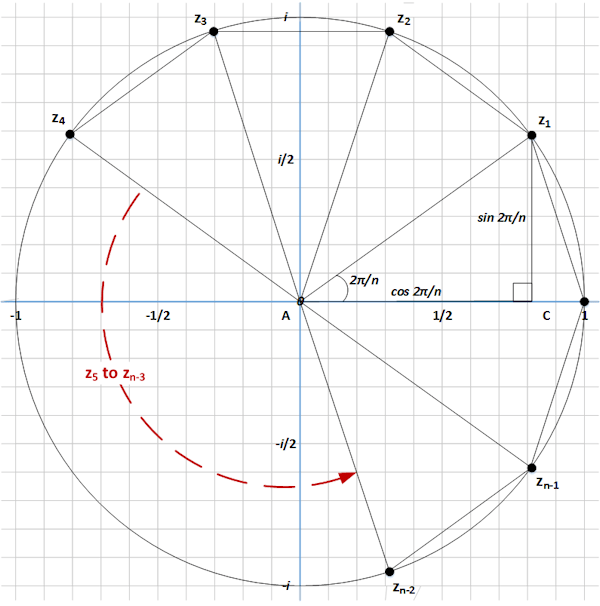Compared to our generic example, we can see that the hypotenuse is of length 1 (a = 1), that θ = 2π/n and that b and c are respectively the Real and Imaginary parts of our suggested root. If we label this root z1, then:

sin(2π/n) = c

and

cos(2π/n) = b

So we can write that:

z1 = cos(2π/n) + i sin(2π/n)

We have now constructed z1 as a candidate nth root of unity. Is it an actual root?

To determine this, we will start with an equation discovered by Euler:

eiθ = cos θ + i sin θ , 

Again we can start by employing a property of powers:

(eiθ)n = eiθn

So, replacing the powers of e with the relevant Trigonometric equivalents from Euler’s Formula, we get:

(cos θ + i sin θ )n = cos nθ + i sin nθ

This result is called De Moivre’s Formula and we will be using it twice in this Chapter.

First of all, if we replace the generic θ in De Moivre’s Formula with the specific 2π/n that we calculated as the angle between the vertices of our n-gon, then we get:

(cos(2π/n) + i sin(2π/n) )n = cos(n2π/n) + i sin(n2π/n)

Cancelling out the ns that appear in both numerators and denominators, this becomes:

(cos(2π/n) + i sin(2π/n) )n = cos(2π) + i sin(2π)

Evaluating the right-hand side (noting that cos 2π = 1 and sin 2π = 0), we get:

(cos(2π/n) + i sin(2π/n))n = 1

The expression that we are raising to the power n on the left-hand side is precisely our definition of our proposed first nth root of unity not equal to 1, z1. We have just shown that z1n = 1. This means that it is indeed an nth root of unity. So our initial geometrical insight has not let us down .

However we have only shown that the first vertex of our n-gon is an nth root of 1. What about the others?

Well we can also see that each vertex is created by adding a further 2π/n to the initial angle. So the location of the second vertex is:

(cos(2π/n + 2π/n) + i sin(2π/n + 2π/n)) =

(cos(2 × 2π/n) + i sin(2 × 2π/n))

and more generically the mth vertex is:

(cos(2mπ/n) + i sin(2mπ/n)

Again employing De Moivre’s Formula we can see that:

(cos(2mπ/n) + i sin(2mπ/n))n = cos(2mnπ/n) + i sin(2mnπ/n) =

cos(2mπ) + i sin(2mπ)

Both cosine and sine are periodic functions, where the period equates to one trip round a circle (a distance of 2π). So cos(2mπ) = 1 and sin(2mπ) = 0 for all m ∈ ℤ. This observation means that:

(cos(2mπ/n) + i sin(2mπ/n))n = 1 for all m ∈ ℤ

and we have thus shown that all of the vertices of our n-gon are nth roots of unity and the proof backing up our Geometrical hunch is complete.

From Trigonometry to Group Theory…

Having established this rather remarkable link between equations and geometry (something we will spend more time on in the next Chapter), it is worth also commenting on something else that might otherwise have slipped by unnoticed. As well as a further strengthening the aforementioned link, this observation also forges a new one with something which should be more familiar.

The first root different from 1 is as we have constructed above:

z1 = cos(2π/n) + i sin(2π/n)

Let’s think about z12, again using De Moivre’s Formula, we can see that:

(cos(2π/n) + i sin(2π/n))2 = cos(4π/n) + i sin(4π/n)

But the term on the right is precisely the next nth root on our list, z2.

Equally:

(cos(2π/n) + i sin(2π/n))3 = cos(6π/n) + i sin(6π/n) = z3

It is obvious that z1m = zm so that all n of our nth roots of unity can be generated by taking powers of the first one. This means that we can write our set of such roots as:

{1, z1, z12, … , z1n-1}

We already know that z1n = 1, so powers of z1 cycle round on themselves. Indeed what we have just shown is that the nth roots of unity form a Group of size n which is isomorphic to Cn, the Cylcic Group of order n, which is in turn isomorphic to ℤn, the integers under addition Modulo n.

We can rewrite our diagram above to reflect this approach (in this I have labelled the first anticlockwise nth root of unity as just z rather than z1, the logic remains the same):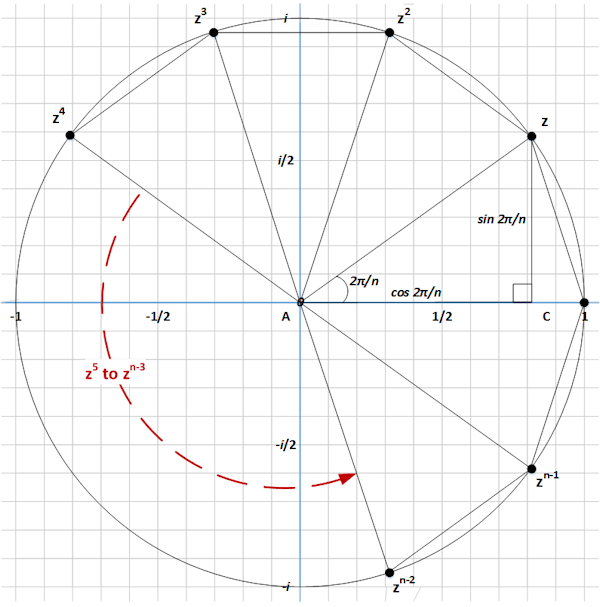In some mysterious way, the roots of a particular set of polynomials, xn – 1 = 0, are the same as the rotational symmetries of an n-gon, which are the same as a property of addition of Integers. Welcome to the way that Group Theory worms its way in to many different areas, or alternatively in which it provides a general and abstract foundational mathematics which can underpin many more concrete structures.

We have found another deep and beautiful result by looking at some specific Polynomials and their roots. Once more, can we extend this finding to develop an understanding of all Polynomials and whether or not they too may relate to Group Theory? It is this question which we will look to address in the next Chapter.

 Concepts Introduced in this Chapter Polynomial An equation of the form: anxn + an-1xn-1 + … + a2x2 + a1 + a0 = 0 Which is to be solved for the unknown, x. The ai, with i ranging from 0 to n, are called the coefficients of the equation. Polynomial Roots A polynomial whose highest power of x is n (i.e. xn) will have n roots. Complex numbers of the form a + ib are needed to solve many Polynomials. nth Roots of Unity Solutions of the equation: xn – 1 = 0 When plotted on the Complex Plane, these are found at the vertices of a regular n-gon, inscribed within a unit circle of radius 1, centred at the origin and with the first vertex lying at 1. Radians An alternative way to measure angles. 360° = 2π radians. Radians are equivalent to the portion of a circle of unit radius that is swept out by an angle and are a more convenient unit of measure for most Mathematical formulae. Powers of Roots of Unity If you square, then cube, then raise to the power 4, etc. the first nth of unity not equal to 1 (or indeed any of the other roots not equal to 1) you will generate all of the other roots, showing that this set forms a cyclic Group of order n.
 Groups Discovered in this Chapter nth roots of unity If we define z1 as the first nth root of unity (moving anticlockwise) which is not 1, then the set {1, z1, z12, … , zn-2n-2, zn-1n-1} covers all other nth roots of unity and forms a Group isomorphic to both Cn and ℤn
 < ρℝεν | ℂσητεητs | ℕεχτ >

Chapter 11 – Notes

  Anything to the power 0 is equal to 1.  There is a reason that Geometry uses diagrams, the prose version can be rather turgid!  The reader is invited to multiply out the two brackets if they would like to check this.  We don’t need the tangent (tan) here, but it feels strange leaving it out.  Save for the earlier reference to Euler’s identity that is mentioned again in the next note, we have come this far without mentioning arguably the most important number in Mathematics, Euler’s Number or e. This margin is too narrow to contain even a brief review of e and its properties, beyond of course the formulae of Euler and De Moivre which appear in this Chapter. We will however meet e again in later Chapters of this book.  We met a special case of this, Euler’s Identity, in Chapter 7, this results if we let θ have the value π, then: eiπ = cos π + i sin π = -1 Euler proved his formula by considering at infinite series expansions for each of ex, cos x and sin x.  A caveat that this is not always the case as per the counter-example offered by The Triangle Paradox.
 Text: © Peter James Thomas 2016-18. Images: © Peter James Thomas 2016-17, unless stated otherwise. Published under a Creative Commons Attribution 4.0 International License.